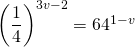Chapter 11: Functions

# 11.4 Exponential Functions

As our study of algebra gets more advanced, we begin to study more involved functions. One pair of inverse functions you will look at are exponential and logarithmic functions.

Exponential functions are functions in which the variable is in the exponent, such as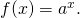Solving exponential equations cannot be done using the techniques used prior. For example, if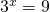, one cannot take the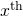root of 9 because we do not know what the index is. However, if you notice that 9 is 32, you can then conclude that, if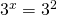, then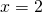. This is the process is used to solve exponential functions.

If the problem is rewritten so the bases are the same, then the exponents must also equal each other.

Example 11.4.1

Solve for the exponent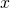in the equation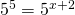.

Since the bases for these exponents are the same, then the exponents must equal each other. Thus: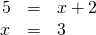Generally,  manipulating the bases on each side of an exponential function to make them equal. These types of problems are as follows:

Example 11.4.2

Solve for the exponentin the equation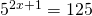.

Since the bases for these exponents are not equal, then the first challenge is to find the lowest common base. For this problem, 125 is the same as 53.

Therefore, the equation is rewritten as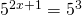. Thus: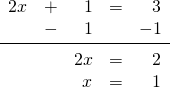Example 11.4.3

Solve for the exponentin the equation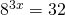.

Finding the common base is a bit more complicated for this problem, but this issue is easily resolved if terms are reduced to their prime factorization of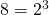and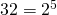. Use this to rewrite the original equation as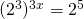.

With identical bases, now solve for the exponents: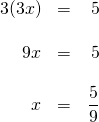Example 11.4.4

Solve for the exponentin the equation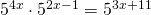.

Since the bases already equal each other, simplify both sides before beginning to solve this problem.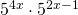reduces to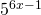, and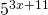is already reduced.

With the bases simplified, now solve: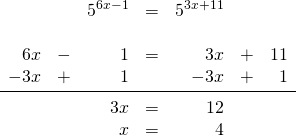Example 11.4.5

Solve for the exponentin the equation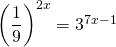.

First, since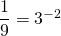, the common base is 3. Rewriting the equation in the base of 3 yields: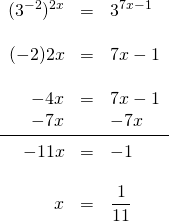# Questions

Solve each equation.

1.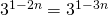2.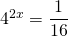3.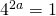4.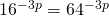5.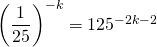6.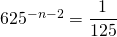7.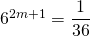8.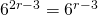9.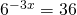10.11.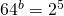12.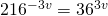13.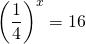14.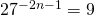15.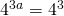16.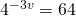17.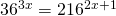18.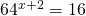19.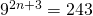20.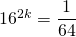21.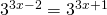22.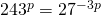23.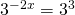24.25.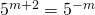26.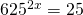27.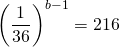28.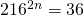29.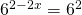30.